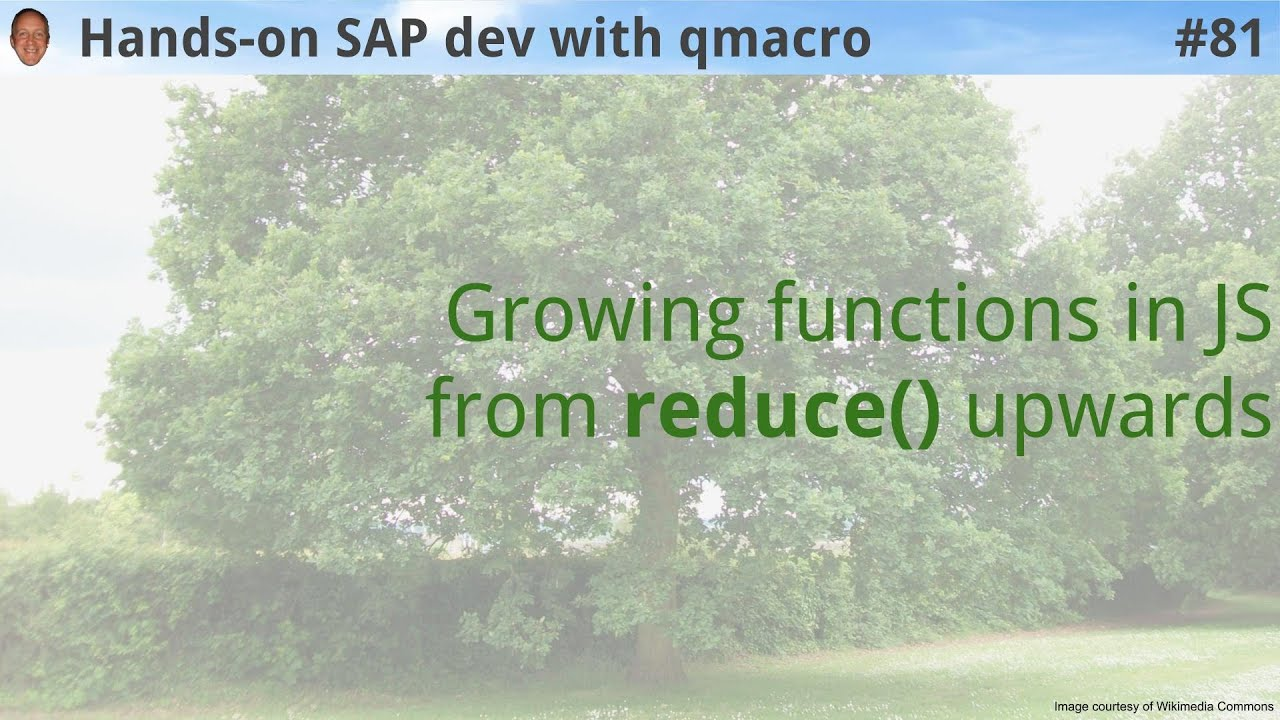# Understanding jq's reduce function

It took me a bit of time to get my head around jq's reduce function. In this post I show how it relates to the equivalent function in JavaScript, which helped me understand it better, and might help you too.

The concept of the reduce function generally is a beautiful thing. I've written about reduce in previous posts on this blog:

Being a predominantly functional language, the fact that `jq` has a reduce function comes as no surprise. However, its structure and how it is wielded is a little different from what I was used to. I think this is partly due to how `jq` programs are constructed, as pipelines for JSON data to flow through.

I decided to write this post after reading an invocation of `reduce` in an answer to a Stack Overflow question, which had this really interesting approach to achieving what was desired:

``reduce ([3,7] | to_entries[]) as \$i (.; .[\$i.key].a = \$i.value)``

Because my reading comprehension of `jq`'s `reduce` was a little off, I found this difficult to understand at first. But now it's much clearer to me.

## The manual entry for reduce

When I first read the entry for `reduce` in the `jq` manual, I found myself scratching my head a little. This is what it says:

The reduce syntax in jq allows you to combine all of the results of an expression by accumulating them into a single answer. As an example, we'll pass [3,2,1] to this expression:

``reduce .[] as \$item (0; . + \$item)``

For each result that .[] produces, . + \$item is run to accumulate a running total, starting from 0.

## Invoking the example

In case you're wondering, the complete invocation, supplying the array `[3,2,1]`, could be done in a number of ways, depending on your preference. Here are two examples:

Passing in the array as a string for `jq` to consume via STDIN:

``echo [3,2,1] | jq 'reduce .[] as \$item (0; . + \$item)'``

Using `jq`'s `-n` option, which tells `jq` to use 'null' as the single input value (effectively saying "don't expect any input") and then using a literal array within the `jq` code:

``jq -n '[3,2,1] | reduce .[] as \$item (0; . + \$item)'``

## Examining the pieces in JavaScript

Regardless of how it is invoked, I wanted to work out which bit of the `reduce` construct did what. I did so by relating the structure to what I was more familiar with - the `reduce` function in JavaScript, which, if we were to do the equivalent of the above, would look like this:

``[3,2,1].reduce((a, x) => a + x, 0)``

So here we have:

• the array of values that we're processing with `reduce` `[3,2,1]`
• the call to the `reduce` function itself, with two things passed to it:
• the anonymous function `(a, x) => a + x` that implements how we want to reduce over the list, often referred to as the "callback" function
• the starting value `0` for the accumulator

If you want to understand each of these parts better, take a quick look at F3C Part 3 - Reduce basics.

When the line of JavaScript above is processed, the `reduce` function first determines the initial value of the accumulator, which is `0` here (the second of the two parameters passed to it). Then it works through the array, calling the anonymous function for each item, and passing:

• the current value of the accumulator (which is `0` for the first item), received in parameter `a`
• the value of the array item being processed (which will be `3` the first time, then `2`, then `1`), received in parameter `x`

Whatever that function produces (which in this case is the value of `a + x`) becomes the new accumulator value, and the process continues with the next array item, and so on.

The final value of the accumulator is the final value of the reduction process (the `reduce` function can produce any shape of data, not just scalar values, but that's an exploration for another time).

## Relating it to jq's reduce

So how do we interpret the `reduce` construct in `jq`? Let's see, this is what we're looking at:

``reduce .[] as \$item (0; . + \$item)``

If we modify the `\$item` variable name so it's `\$x`, we can more easily pick out the component parts and relate them to what we've just seen:

``reduce .[] as \$x (0; . + \$x)``

Here we see:

• `.[] as \$x` is the reference to the array we want to process with `reduce` (remember, this will pass through whatever list is piped into this filter) and the the variable (`\$x`) that will be used to represent each array item as they're processed
• the `0` is the starting value for the accumulator
• the `. + \$x` is the expression that is executed each time around (equivalent to `a + x` in the JavaScript example), where the accumulator is passed in to it (i.e. the accumulator is the `.` in the expression)

And the final value of the `. + \$x` expression, i.e. the final value of the accumulator, is what then represents the output of this `reduce` function invocation.

That's pretty much it!

I found this post from Roger Lipscombe useful for my understanding too: jq reduce.

Finally, you may also be interested in this live stream recording on what `reduce` is and how it can be used to build related functions:

HandsOnSAPDev Ep.81 - Growing functions in JS from reduce() upwards